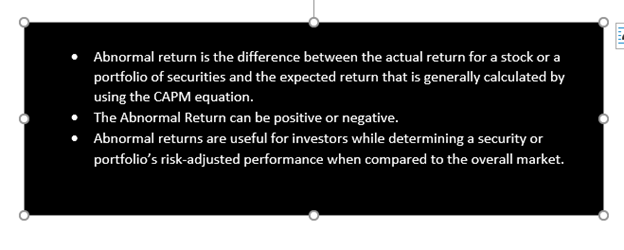# Abnormal Return

• Updated on

What is Abnormal Return?

Abnormal Return, also refer as Excess Return is the unexpected large profit or losses generated by securities or stocks over a specified period. Abnormal returns is a variance between the actual return for a stock or a portfolio of securities and the expected return that is determined by using the Capital Asset Pricing Model (CAPM) equation.

The Abnormal Return can be positive or negative. The Positive abnormal return occurs when the expected return is lower than the actual return, while negative abnormal return or losses occurs when the expected return is greater than the actual return. Abnormal Return helps investors to determine risk-adjusted performance and it should not be confused with alpha or excess return earned by actively managed investments. It can be produced by change, due to unforeseen event, external factors or as the result of bad actors.Understanding Abnormal Return

Abnormal returns are useful for investors while determining a security or portfolio’s risk-adjusted performance when compared to the overall market or benchmark index and it may help in identifying a portfolio manager’s skill on a risk-adjusted basis. It also helps in determining if investors received adequate return for the risk assumed.

For example, receiving 30% in an asset that is expected to average 10% each year would generate positive abnormal return of 20%. In case, the actual return was 5% the negative abnormal return would be 5%.

How to calculate abnormal return

To calculate the Expected return, the Capital Asset pricing model (CAPM) is used.

Equation for the Capital Asset Pricing model:

Er = Rf + β (Rm - Rf)

Here,

Er = Expected return in security or portfolio

R= risk-free rate, generally the rate of a government security or savings deposit rate

β= risk coefficient of the security or the portfolio as compared to the market

Rm= Return on the market

Once we get the expected return, subtract it from the actual return to determine the Abnormal return.

Abnormal return = Actual return – Expected return

In case, the security or portfolio has underperformed the expectations the abnormal return will be negative. Otherwise it will be positive or zero.

As per the prudent approach, it’s better to consider risk-adjusted return as portfolio manager may invest in high risk investment to generate abnormal return. In case of multiple periods, look for the standardized returns to know if the portfolio is constantly beating the benchmark, if it is then it indicates that manager has made investment in a better stocks and the standard deviation of the abnormal return will be lower.

Cumulative Abnormal Return (CAR)

Cumulative abnormal return is the sum total of all the abnormal returns over a given period of time that enable investors to determine the performance of an asset or security over a particular period of time, as abnormal return are usually calculated over a small window of time and compounding it daily gives biased results. CAR may be used to determine the effect of buyouts, lawsuits and other events have on stock prices and it is also used to know the reliability of asset pricing models in determining the expected performance.

The capital asset pricing model (CAPM) is framework used to determine the expected return of an asset, security or a portfolio, based on the risk-free rate of return, expected market return and beta. After determining the expected return, the assumption of abnormal return is determined by subtracting the expected return from the realized return.Importance of abnormal return

• Performance Attribute Metric

Abnormal return gets affected by the stock selection of the portfolio manager, so it can be used to measure the performance of manager as compared to the appropriate benchmark.

• A check on Harmful Divergence

As abnormal return can be negative if expected return is higher than the actual return. If negative abnormal return occurs frequently, it acts as an alarm for reducing the divergence from the benchmark index as it indicate poor stock selection.

• Thorough quantitative analysis

The capital asset pricing model (CAPM) is used to determine abnormal return, it involves the use of regression analysis to calculate beta and a thorough analysis of the past return number of the market index, to produce number with greater predictive power.

• Time Series Analysis

Cumulative abnormal return (CAR) analysis the effect of corporate actions such as stock split or dividend payout on the prices and return on the assets, security or portfolio. Further, it also helps in determining the effect of external events such as events on which certain corporate liabilities are contingent.

##### Top ASX Listed Companies

Definition:
We use cookies to ensure that we give you the best experience on our website. If you continue to use this site we will assume that you are happy with it. OK